# AQA A Level Chemistry复习笔记 6.2.4 Formation of Coloured Ions

### Colour in Transition Metal Ions

• Most transition element complexes are coloured
• The transition metal ions can be identified by their colour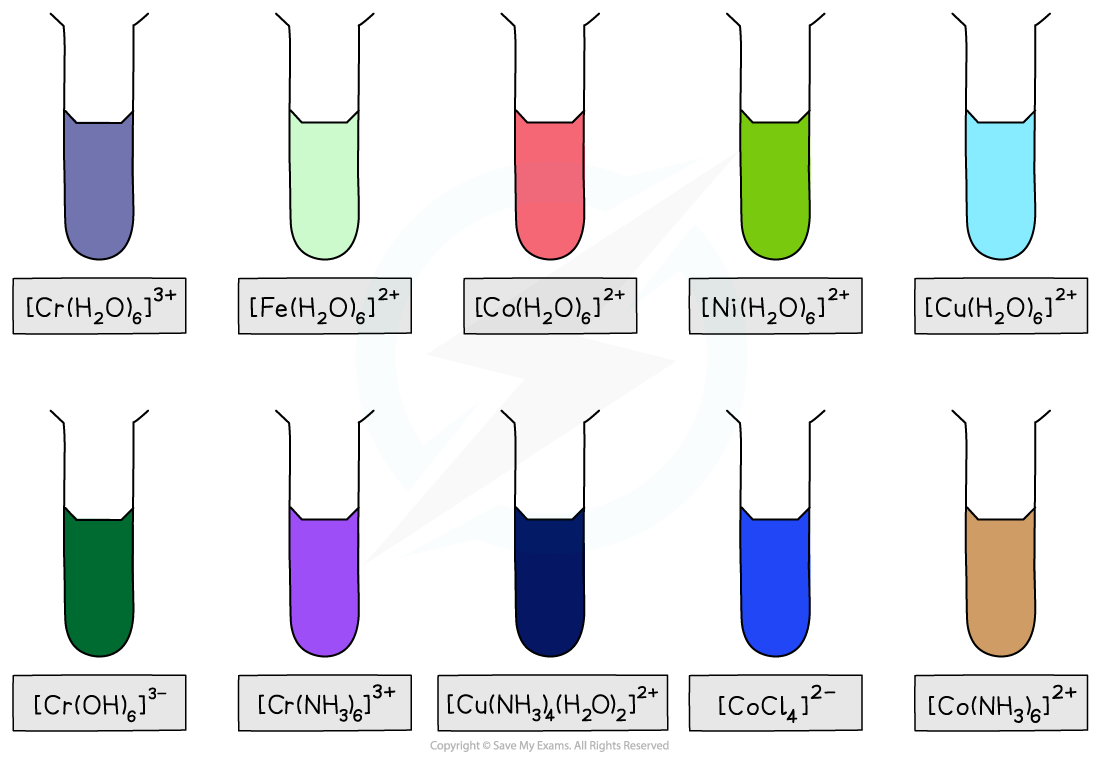The large variety of coloured compounds is a defining characteristic of transition metals

• A transition element complex solution which is coloured, absorbs wavelengths of light in the visible light region of the electromagnetic spectrum
• The observed colour is the complementary colour which is made up of wavelengths of light that are transmitted or reflected
• For example, copper(II) ions absorb light from the red end of the spectrum
• The complementary colour observed is therefore blue-green (cyan)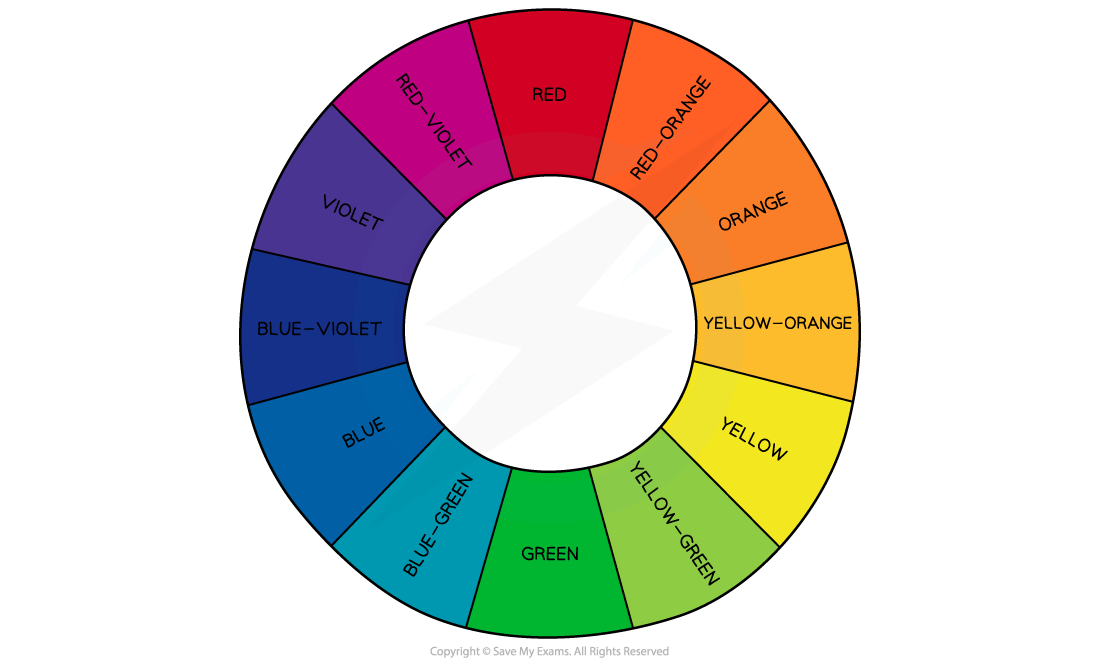The colour wheel showing complementary colours in the visible light region of the electromagnetic spectrum

#### Electron promotion

• In an isolated transition element ion (which is not bonded to any ligands), all of the 3d orbitals are equal in energy
• The term used is degenerate

• However, when ligands are attached to the central metal ion through dative covalent bonds, these orbitals are split into two sets of non-degenerate orbitals
• The difference in energy between these two sets of orbitals is ΔE
• When light shines on a solution containing a transition element complex, an electron will absorb this exact amount of energy (ΔE)
• The amount of energy absorbed can be worked out by the equation:

ΔE = h x v

h = Planck's constant (6.626 x 10-34 m2 kg s-1)

v = frequency (Hertz, Hz or s-1)

• The electron absorbs light energy that then excites it from a 3d orbital of lower energy to one of higher energy (excited state)
• This is also called electron promotion

• The other frequencies of light which are not absorbed combine to make the complementary colour
• The diagram below shows an example of electron promotion in an octahedral complex of a nickel(II) Ni2+ ion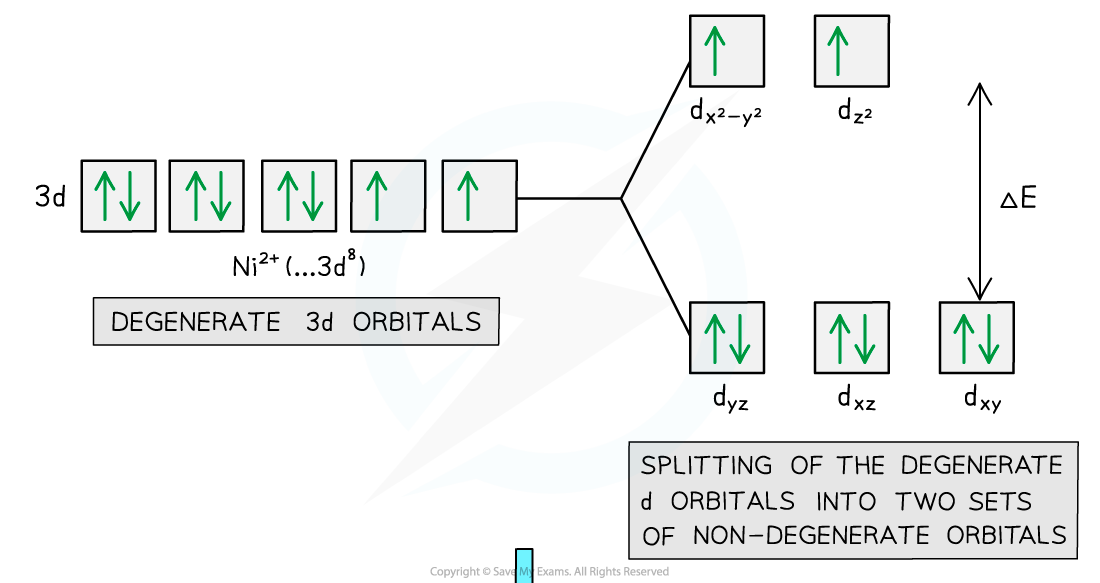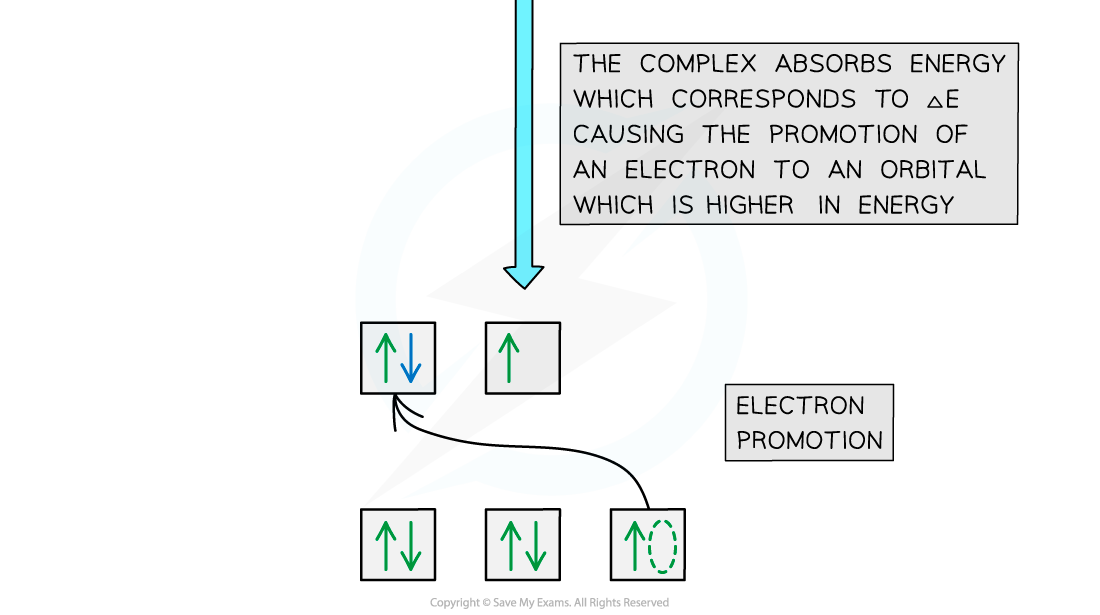Electron promotion in a Ni(II) complex when light shines on the solution

### Changes in Colour

• Transition element complexes absorb the frequency of light which corresponds to the exact energy difference (ΔE) between their non-degenerate d orbitals
• The frequencies of light which are not absorbed combine to make the complementary colour of the complex
• It is the complementary colour which is transmitted and observed
• However, the exact energy difference (ΔE) is affected by factors such as
• the type of ligand
• the coordination number
• the oxidation state of the transition metal ion

#### Type of ligand

• Different ligands will split the d orbital by a different amount of energy
• This depends on the repulsion that the d orbital experiences from these ligands
• Therefore, the size of ΔE and thus the frequency of light absorbed by the electrons will be slightly different
• As a result, a different colour of light is absorbed by the complex solution and a different complementary colour is observed
• This means that complexes with similar transition elements ions, but different ligands, can have different colours
• For example, the [Cu(H2O)6]2+ complex has a light blue colour
• Whereas the [Cu(NH3)4 (H2O)2]2+ has a dark blue colour
• Despite the copper ion having an oxidation state of +2 in both complexes
• This is evidence that the ligands surrounding the complex ion affect the colour of the complex

#### Coordination number

• The coordination number also influences the strength of the metal ion-ligands interactions
• Changing the coordination number generally involves changing the ligand as well, so it is a combination of these factors that alters the strength of the interactions

#### Oxidation State

• The strength of the attraction between the transition metal ion and the electrons pairs in the dative covalent bonds can vary depending on the effective nuclear charge of the metal ion
• Manganese(II) and iron(III) have the same electronic configuration, which is 1s22s22p63s23p63d5
• [Mn(H2O)6]2+ appears pink because it absorbs in the green region of the spectrum, following the principle of complementary colours
• The higher effective nuclear charge on aqueous [Fe(H2O)6]3+ means it has a stronger interaction with the ligands so it absorbs in the blue higher energy end of the spectrum and appears orange in colour
• When the same metal is in a higher oxidation state that will also create a stronger interaction with the ligands
• If you compare iron(II) and iron (III), [Fe(H2O)6]2+ absorbs in the red region and appears green, but [Fe(H2O)6]3+absorbs in blue region and appears orangeThe visible part of the electromagnetic spectrum shows the relationship between colour and energy. The red end of the spectrum is lower energy and the blue end is higher energy

### Visible Light Spectroscopy

• Spectroscopy is the study of the interactions between light and matter
• A simple colorimeter is used to find the concentration of coloured transition metal ion solutions
• The colorimeter passes different frequencies of light through a sample of the solution
• The frequency absorbed is determined by the use of coloured filters and a detector
• A filter is chosen matching the part of the spectrum where the absorption is strongest
• This means choosing a filter that is the complementary colour to the colour of the solution
• For example, a blue solution will absorb red, and therefore a red filter is used, so that only red light passes through the solution and maximum absorption occurs

• Some of the light is absorbed by the solution and the rest passes through to the detector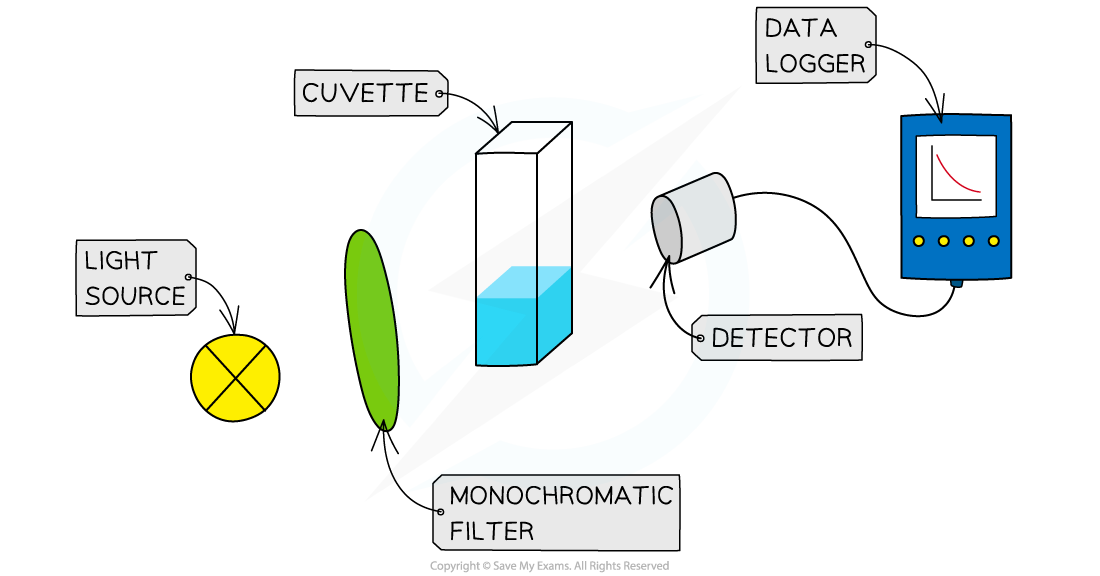The working principles of a visible light colorimeter

• To determine the concentration of a coloured solution first a calibration curve has to be made
• This involves measuring the absorption of a set of standard solutions whose concentration is known
• The values are then plotted on a graph of absorption against concentrationA calibration curve is used to find the concentration of unknown coloured solutions of transition metal ions

• At lower concentrations the absorbance is directly proportional to the concentration of the coloured species so a straight line graph is obtained; this is known as the Beer-Lambert Law
• Once the calibration curve is plotted then the absorbance of any unknown solution of the same complex ion can be measured
• You can then deduce the concentration of the unknown sample by extrapolating from the absorbance to the calibration curve and down to the concentration
• Limitations of visible light spectroscopy can be:
• A very darkly coloured solution can be difficult for a colorimeter to read accurately
• A very pale solution may also be at the limit of the colorimeters sensitivity; however a work around is to use ligand exchange to convert the complex into a more strongly coloured one
• Adding thiocyanate ions to dilute solutions of  [Fe(H2O)6]3+ produces a red coloured complex allowing the analyses of lower concentrations in iron(III

[Fe(H2O)6]3+   (aq)  + SCN- (aq) ⇌  [Fe(H2O)5 SCN]2+ (aq) + H2O (l)

pale orange             colourless            blood-red

#### Exam Tip

You should know the factors that change the colour of transition metal complex ions and be able to write equations to give examples of those changes.